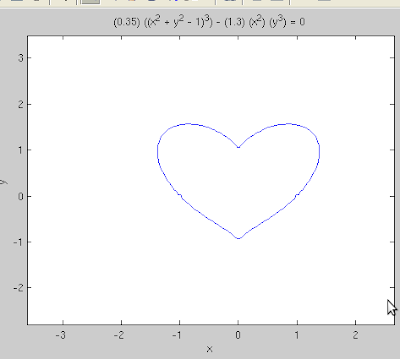## Wednesday, April 28, 2010

### HEART TO HEART

HEART TO HEART

A very interesting mathematical recreation is to conceive coherent drawings using algebraic functions. Bezier curves are the authentic way to generate such computer graphics. Bezier came up with his genius in early 60s while designing automobile parts. Other popular means are Hermite curves and Splines.

Though effective, these means are involved and computationally demanding. However, sometimes by providence we do have sensible shapes made out from simple functions.

PLOTTING THE HEART IN MATLAB

I discuss plotting a heart using simple cardioid, polar plots and algebraic equations

(a) The simplest one

The simplest 'heart curve' is the cardioid. It is not truly a heart, however it looks somewhat similar to one.(b) Polar plot

A polar curve given by;gives a near perfect heart !The Matlab command line is;

>> ezpolar('((sin(t)*sqrt(abs(cos(t))))*(sin(t) + (7/5))^(-1)) - 2*sin(t) + 2')

(c) Algebraic Equation

A heart can be made from the implicit algebraic function,However tinkering by adding a factor of (0.35) and (1.3) to the terms makes the heart more aesthetic.The Matlab command is,

>> ezplot('(0.35)*((x^2 + y^2 - 1)^3) - (1.3)*(x^2)*(y^3)');

It is worth noting that for using ezplot t, x and y doesn't have to be defined.

(d) Another Algebraic Equation

#### 3 comments:

Luke Dunn said...

like it ! I believe the cardioid shape has unusual properties as a microphone. the curve points towards the front where the singer or speaker is and the cusp towards the back. there is a reduction of noise pollution originating from any position behind the mike, because the cusp reflects incident sound away.

Arkapravo said...

@Luke : well .... that is another view point .... and really has a 'rythm' to it :) .... dude, I never looked at the cardioid from acoustical point of view !

Mike Croucher said...

A nice post - I like it. For a 3d cardioid in MATLAB check out the following post.

http://www.walkingrandomly.com/?p=455

The image shown is a star but there is code for both a star and a cardioid.

Cheers,
Mike